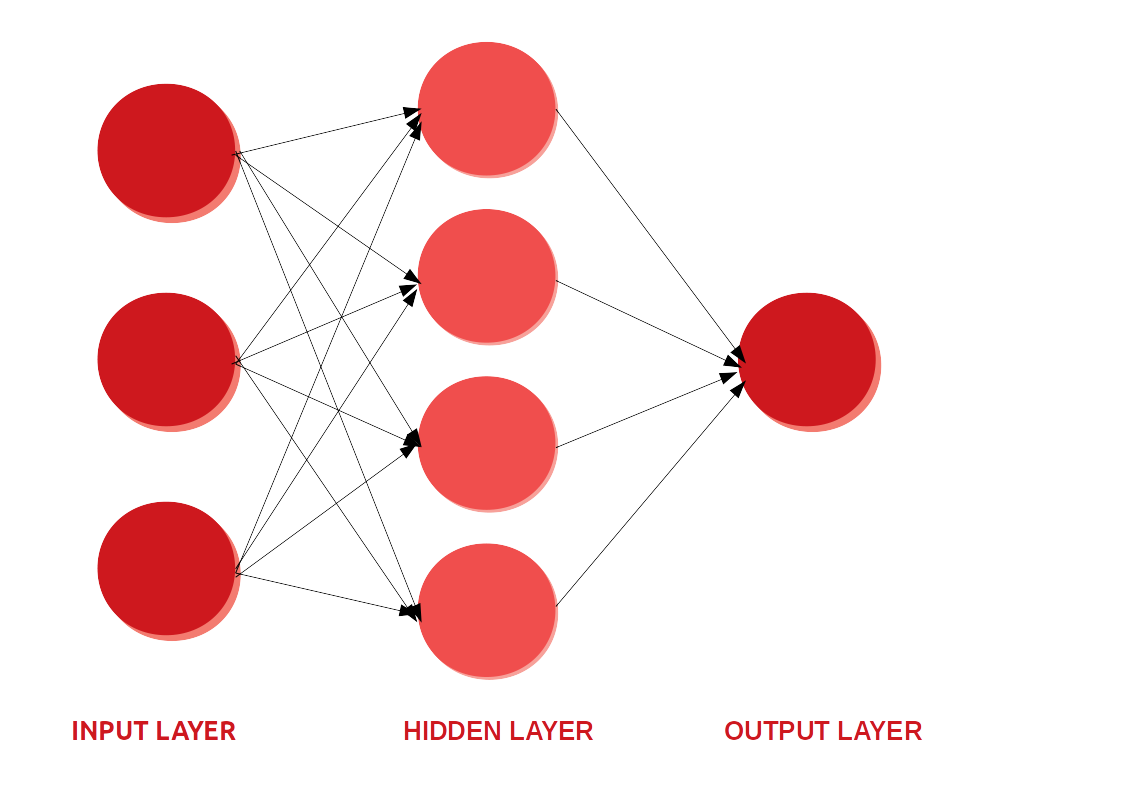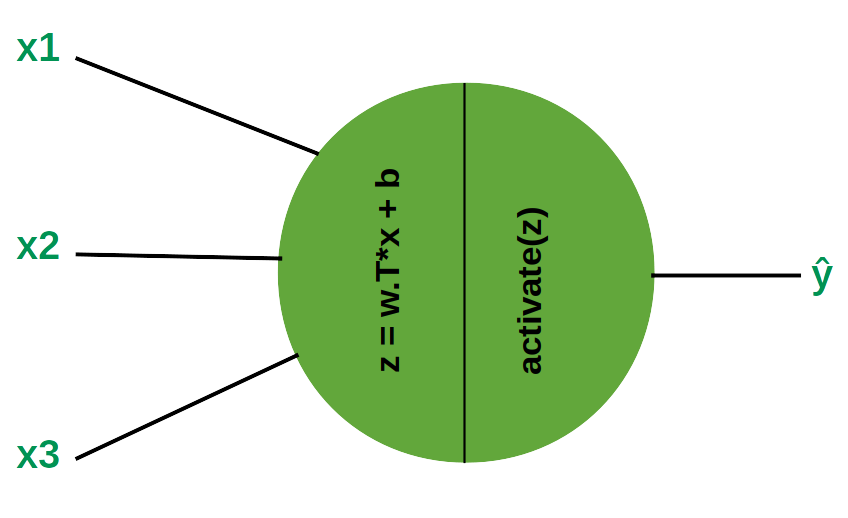# What, Why and Which?? Activation FunctionsWhile studying neural network, we often come across the term — “Activation functions”. What are these activation functions?

### 1. The basic idea how a neural network works —Simple neural network

Neural networks are information processing paradigms inspired by the way biological neural systems process data. It contains layers of interconnected nodes or neurons arranged in interconnected layers. The information moves from the input layer to the hidden layers. In a simple case of each layer, we just multiply the inputs by the weights, add a bias and apply an activation function to the result and pass the output to the next layer. We keep repeating this process until we reach the last layer. Hidden layers fine-tune the input weightings until the neural network’s margin of error is minimal. We would update the weights and biases of the neurons on the basis of the error.

### 2. What is an Activation function?

The activation function is a mathematical “gate” in between the input feeding the current neuron and its output going to the next layer. They basically decide whether the neuron should be activated or not.

Consider a neuron —A Neuron

### 3. Why do we use an activation function ?

If we do not have the activation function the weights and bias would simply do a linear transformation. A linear equation is simple to solve but is limited in its capacity to solve complex problems and have less power to learn complex functional mappings from data. A neural network without an activation function is just a linear regression model.

Generally, neural networks use non-linear activation functions, which can help the network learn complex data, compute and learn almost any function representing a question, and provide accurate predictions.

#### 3.1 Why use a non-linear activation function?

If we were to use a linear activation function or identity activation functions then the neural network will just output a linear function of input. And so, no matter how many layers our neural network has, it will still behave just like a single layer network because summing these layers will give us another linear function which is not strong enough to model data.

Also our activation function should be differentiable.